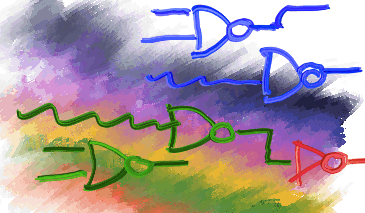The following quiz deals with the subject of Logic Gates and Circuits. Please read the appropriate sections in the text as well as the lecture notes and then answer the questions below. (Go back to homepage.) Which of the following gates generates the truth table shown? ```x y | F ------+---- 0 0 | 0 0 1 | 1 1 0 | 1 1 1 | 0 ```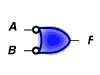The gate function illustrated is an equivalent representation for an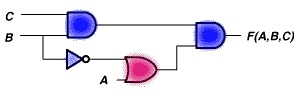Identify the function generated by the logic network illustrated.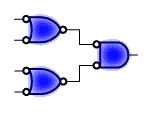Identify the gate elements in the circuit below. In general, a NAND-NAND circuit is equivalent to which of the following circuit types?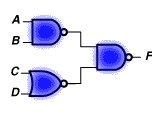Identify that function generated by the logic circuit shown. Integrated logic circuits whose gate complexity ranges between 20 and 200 gates are classified as: Which of the following digital logic families have the lowest propagation delays (i.e. highest speeds)? Which of the following IC digital logic families have the best low-power characteristics?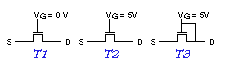Determine the states of the NMOS transistors, given the gate voltages shown below: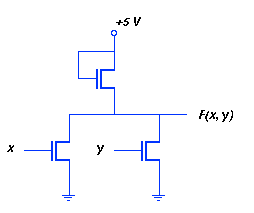Identify the NMOS gate circuit shown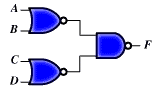Identify that function generated by the logic circuit shown.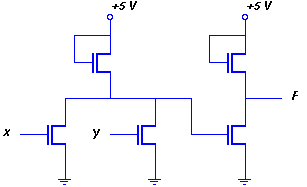Identify the NMOS gate circuit shown.